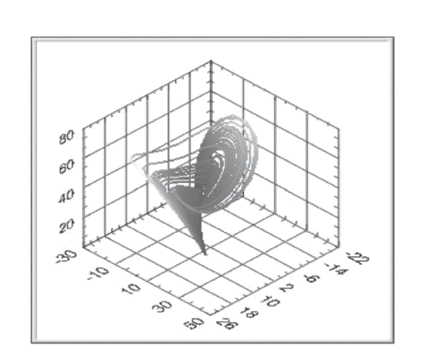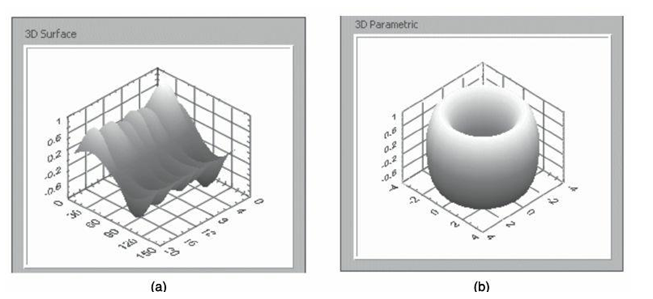# 3d Graphs In Labview

LabVIEW 3D Graphs are used to plot data in three dimensions. Changing the properties of a three-dimensional graph allows you to visualize data in three dimensions. In this post, we’ll show you how to create 3D graphs in LabVIEW.

Rating: 5

6459

## 3D Graphs

For many real-world data sets such as the temperature distribution on a surface, joint time-frequency analysis and the motion of an airplane, you need to visualize data in three dimensions. With the 3D graphs, you can visualize three-dimensional data and alter the way that data appears by modifying the 3D graph properties.

LabVIEW includes the following types of 3D graphs:

• 3D surface graph: Draws a surface in 3D space.
• 3D parametric surface graph: Draws a parametric surface in 3D space.
• 3D curve graph: Draws a line in 3D space.

If you would like to Enrich your career with an Android certified professional, then visit Mindmajix - A Global online training platform: “LabVIEW training” Course.This course will help you to achieve excellence in this domain.

Use the 3D graphs in conjunction with the 3D Graph VIs to plot curves and surfaces. A curve contains individual points on the graph, each point having an x, y, and z coordinates. The VI then connects these points with a line. A curve is ideal for visualizing the path of a moving object, such as the flight path of an airplane. The below Figure shows an example of a 3D curve graph.A surface plot uses x, y, and z data to plot points on the graph. The surface plot then connects these points forming a three-dimensional surface view of the data. For example, you could use a surface plot for terrain mapping. Figure (a) shows examples of a 3D surface graph and Figure (b) shows a 3D parametric surface graph. The 3D graphs use ActiveX technology and VIs that handle 3D representation. When you select a 3D graph, LABVIEW places an ActiveX container on the FRONT PANEL that contains a 3D graph control. LABVIEW also places a reference to the 3D graph control on the BLOCK DIAGRAM. LabVIEW wires this reference to one of the three 3D Graph VIs.Complete the following steps to plot data on a 3D graph.
1. Add a 3D graph to the front panel.
2. Select a POLYMORPHIC instance from the helper VI on the block diagram.
3. Configure the appearance of the graph with the 3D Graph properties. Use the Property Node to configure the appearance from the block diagram. Select 3D Plot Properties from the shortcut menu to configure the appearance with the 3D Plot Properties dialog box.
4. Wire data to each input to graph the plot.
5. Run the VI.Course Schedule
NameDates
LabVIEW TrainingJan 31 to Feb 15
LabVIEW TrainingFeb 04 to Feb 19
LabVIEW TrainingFeb 07 to Feb 22
LabVIEW TrainingFeb 11 to Feb 26
Last updated: 29 January 2023